## Описание презентации Physics of Semiconductor Devices Lecture 4. Generation по слайдам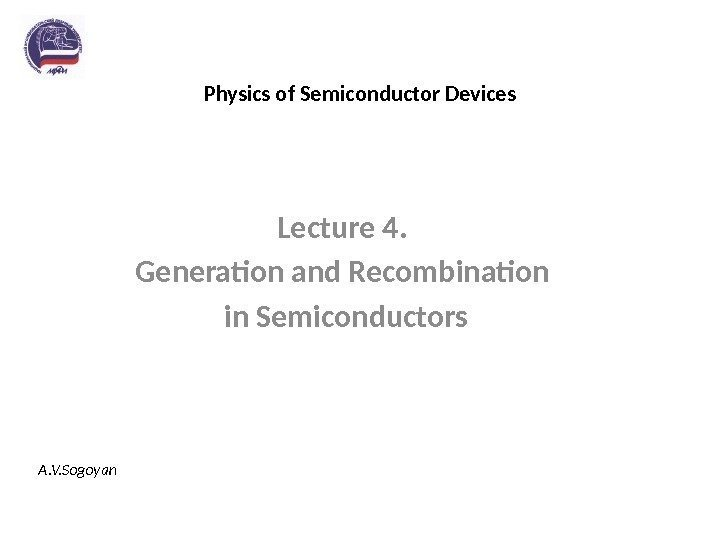Physics of Semiconductor Devices Lecture 4. Generation and Recombination in Semiconductors A. V. Sogoyan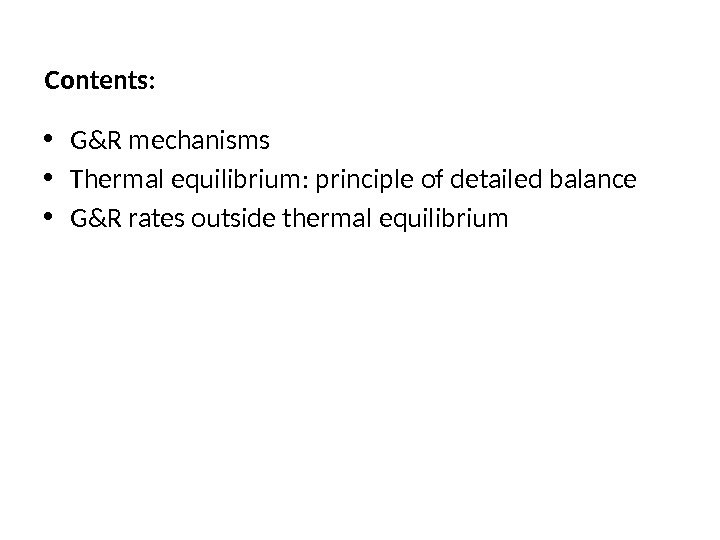Contents: • G&R mechanisms • Thermal equilibrium: principle of detailed balance • G&R rates outside thermal equilibrium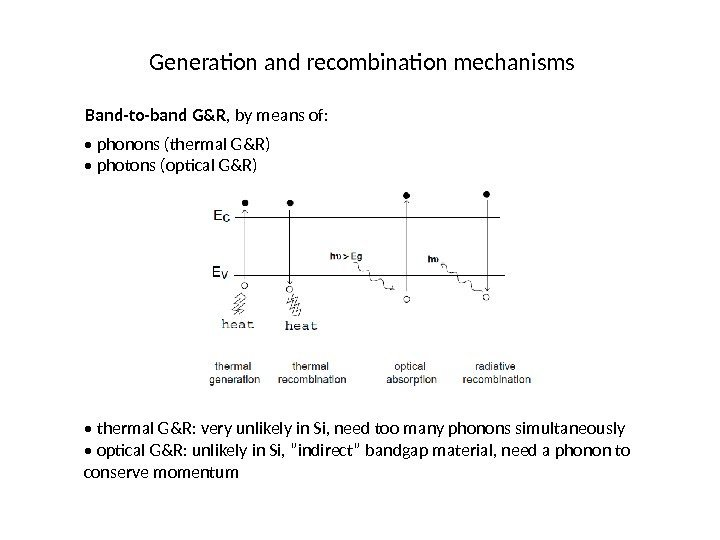Generation and recombination mechanisms Band-to-band G&R , by means of: • phonons (thermal G&R) • photons (optical G&R) • thermal G&R: very unlikely in Si, need too many phonons simultaneously • optical G&R: unlikely in Si, ”indirect” bandgap material, need a phonon to conserve momentum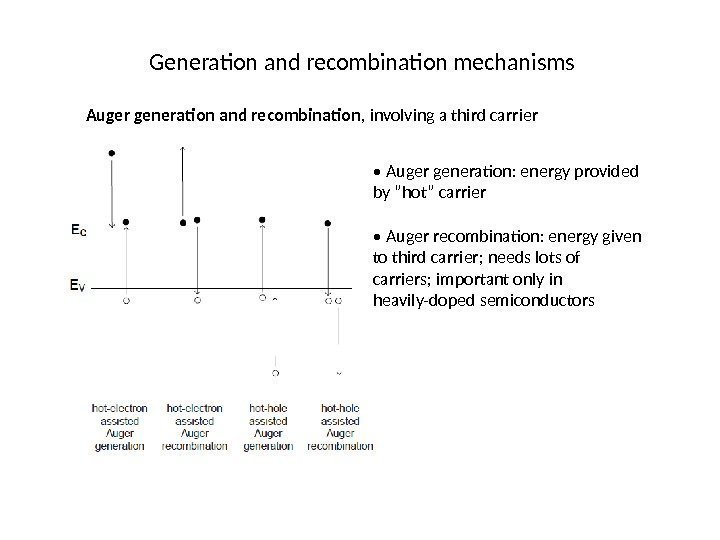Generation and recombination mechanisms Auger generation and recombination , involving a third carrier • Auger generation: energy provided by ”hot” carrier • Auger recombination: energy given to third carrier; needs lots of carriers; important only in heavily-doped semiconductors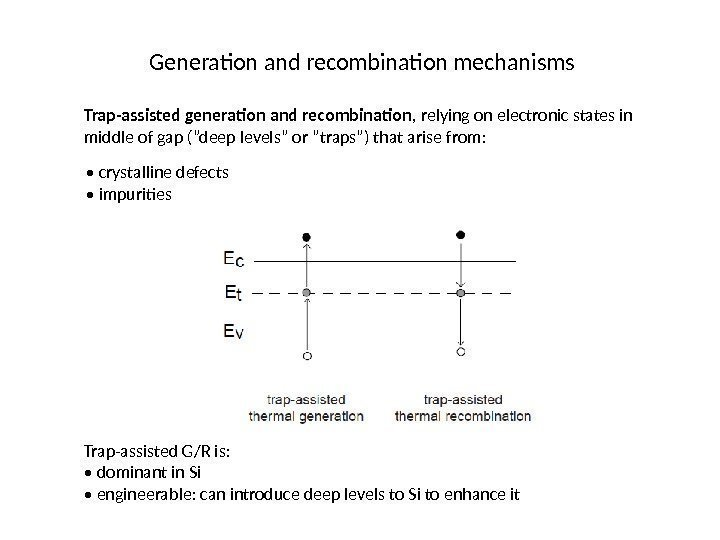Generation and recombination mechanisms Trap-assisted generation and recombination , relying on electronic states in middle of gap (”deep levels” or ”traps”) that arise from: • crystalline defects • impurities Trap-assisted G/R is: • dominant in Si • engineerable: can introduce deep levels to Si to enhance it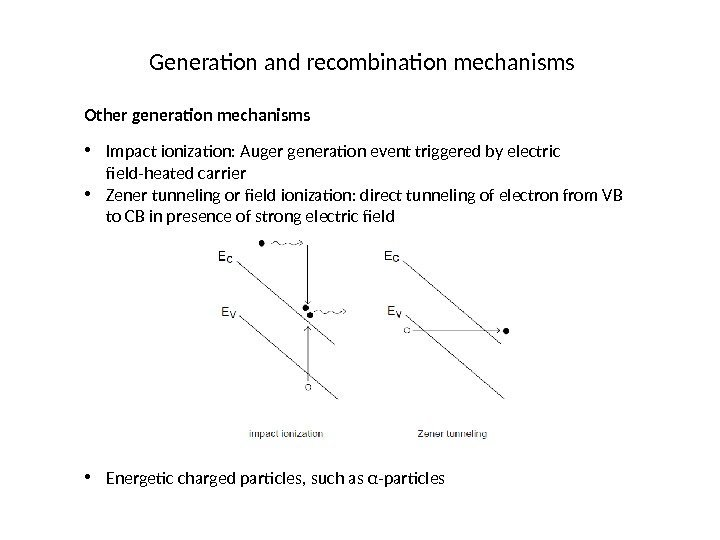Generation and recombination mechanisms Other generation mechanisms • Impact ionization: Auger generation event triggered by electric field-heated carrier • Zener tunneling or field ionization: direct tunneling of electron from VB to CB in presence of strong electric field • Energetic charged particles, such as α-particles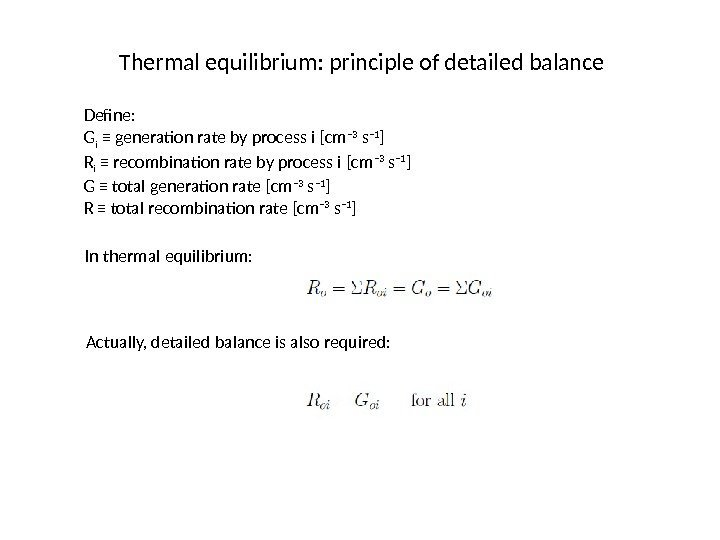Thermal equilibrium: principle of detailed balance Define: G i ≡ generation rate by process i [cm − 3 s − 1 ] R i ≡ recombination rate by process i [cm − 3 s − 1 ] G ≡ total generation rate [cm − 3 s − 1 ] R ≡ total recombination rate [cm − 3 s − 1 ] In thermal equilibrium: Actually, detailed balance is also required: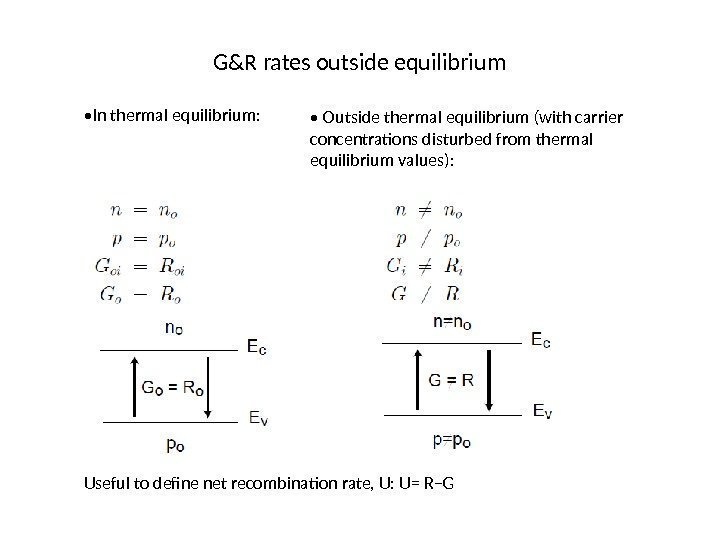G&R rates outside equilibrium • In thermal equilibrium: • Outside thermal equilibrium (with carrier concentrations disturbed from thermal equilibrium values): Useful to define net recombination rate, U: U= R−G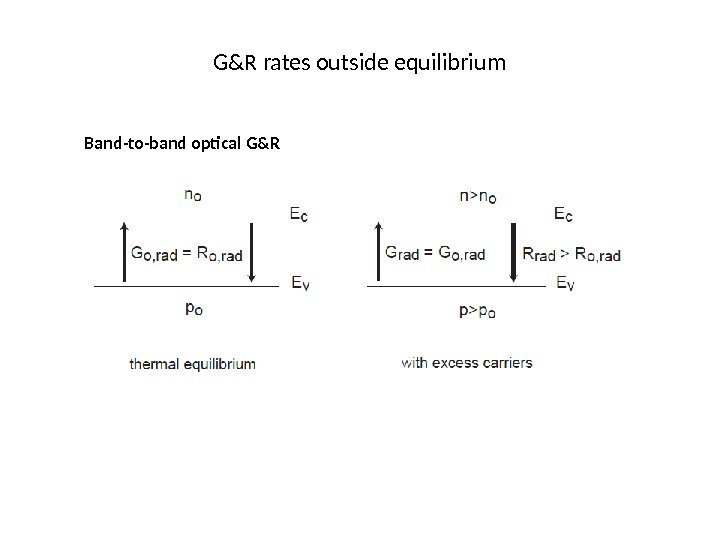G&R rates outside equilibrium Band-to-band optical G&R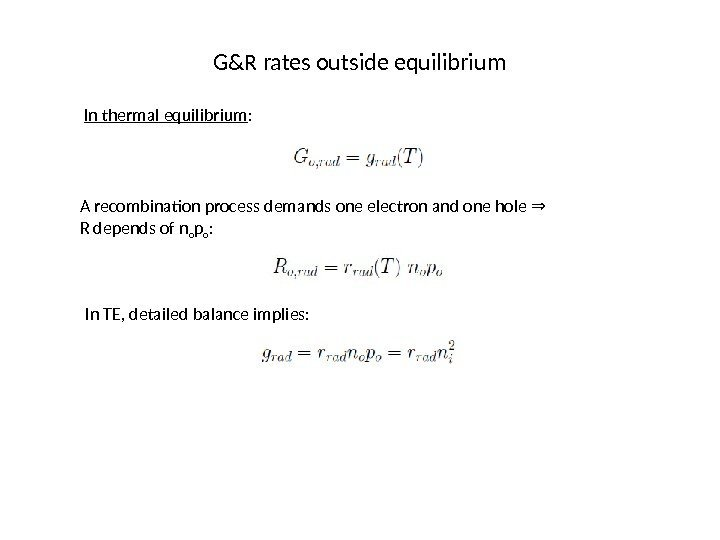G&R rates outside equilibrium In thermal equilibrium : A recombination process demands one electron and one hole ⇒ R depends of n o p o : In TE, detailed balance implies: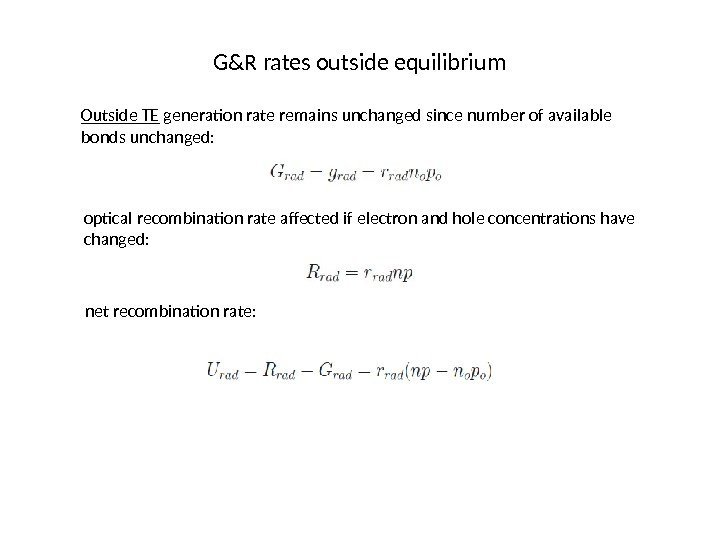G&R rates outside equilibrium Outside TE generation rate remains unchanged since number of available bonds unchanged: optical recombination rate affected if electron and hole concentrations have changed: net recombination rate: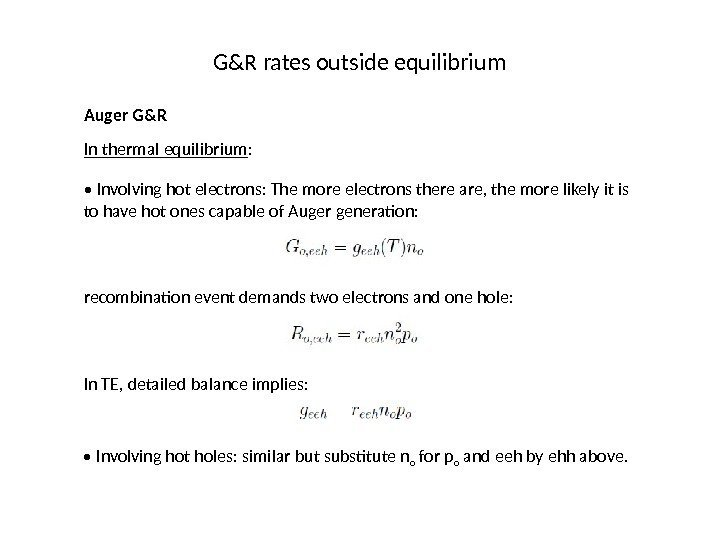G&R rates outside equilibrium Auger G&R In thermal equilibrium : • Involving hot electrons: The more electrons there are, the more likely it is to have hot ones capable of Auger generation: recombination event demands two electrons and one hole: In TE, detailed balance implies: • Involving hot holes: similar but substitute n o for p o and eeh by ehh above.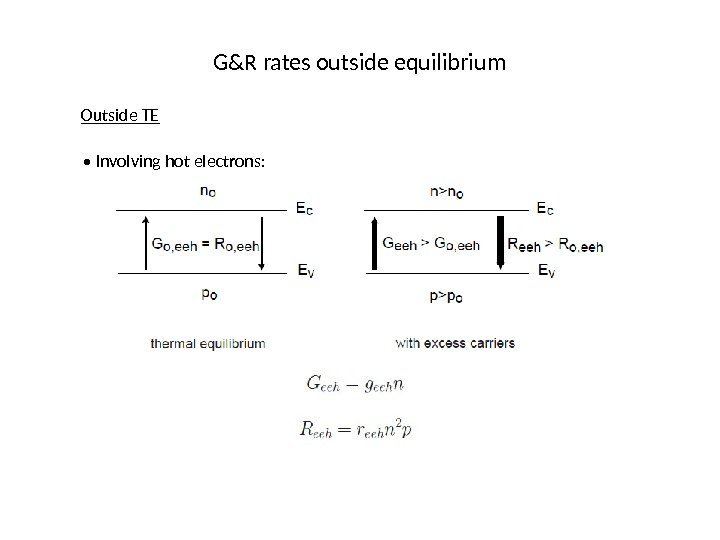G&R rates outside equilibrium Outside TE • Involving hot electrons:G&R rates outside equilibrium If relationship between g eeh and r eeh unchanged from TE: • Involving hot holes, similarly: • Total Auger: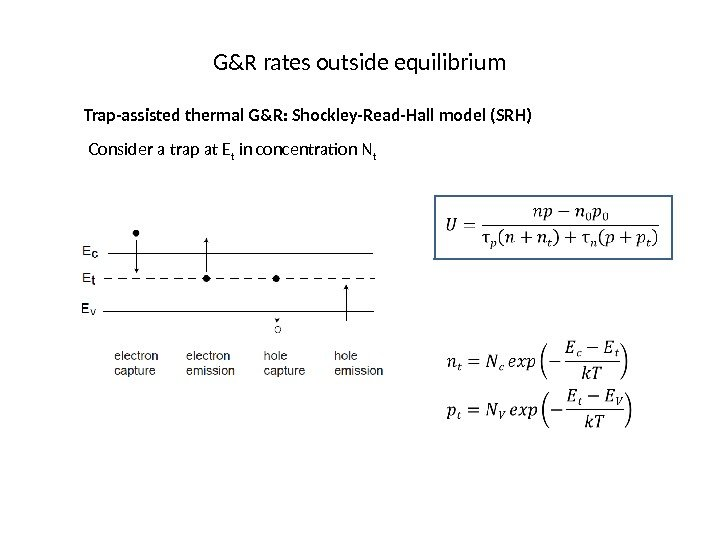G&R rates outside equilibrium Trap-assisted thermal G&R: Shockley-Read-Hall model (SRH) Consider a trap at E t in concentration N t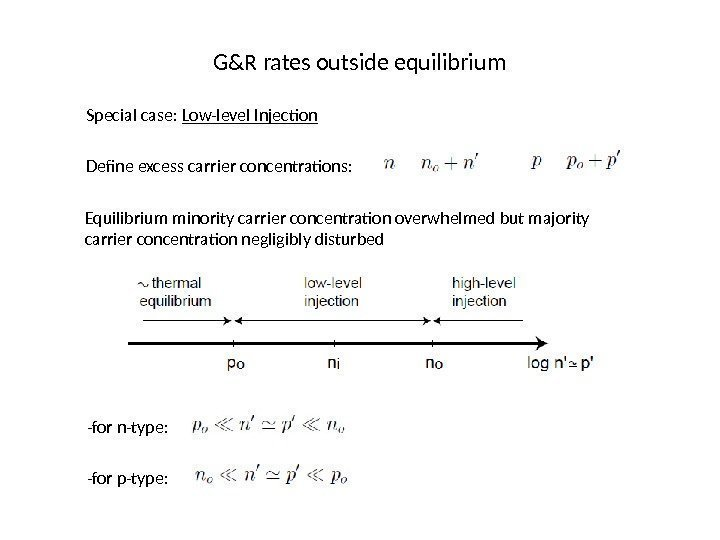G&R rates outside equilibrium Special case: Low-level Injection Define excess carrier concentrations: Equilibrium minority carrier concentration overwhelmed but majority carrier concentration negligibly disturbed -for n-type: -for p-type: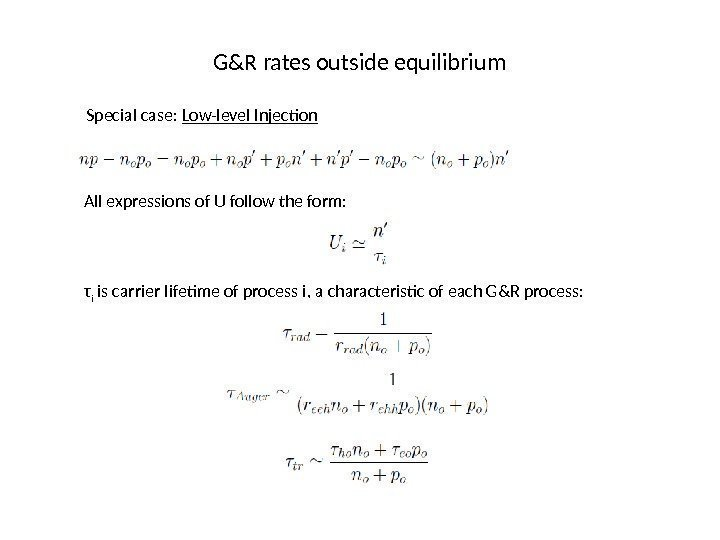G&R rates outside equilibrium Special case: Low-level Injection All expressions of U follow the form: τ i is carrier lifetime of process i, a characteristic of each G&R process: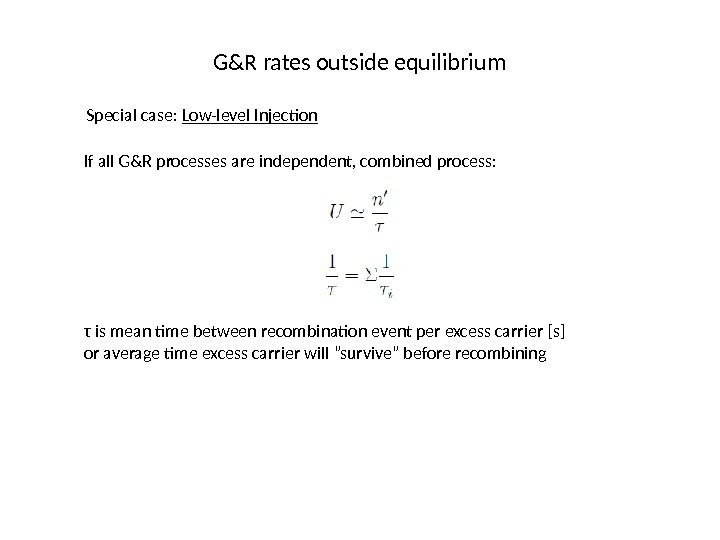G&R rates outside equilibrium Special case: Low-level Injection If all G&R processes are independent, combined process: τ is mean time between recombination event per excess carrier [s] or average time excess carrier will ”survive” before recombining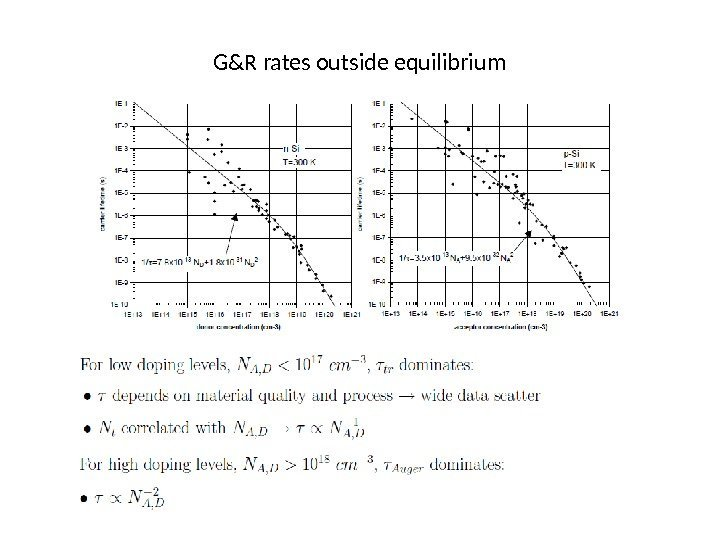G&R rates outside equilibrium